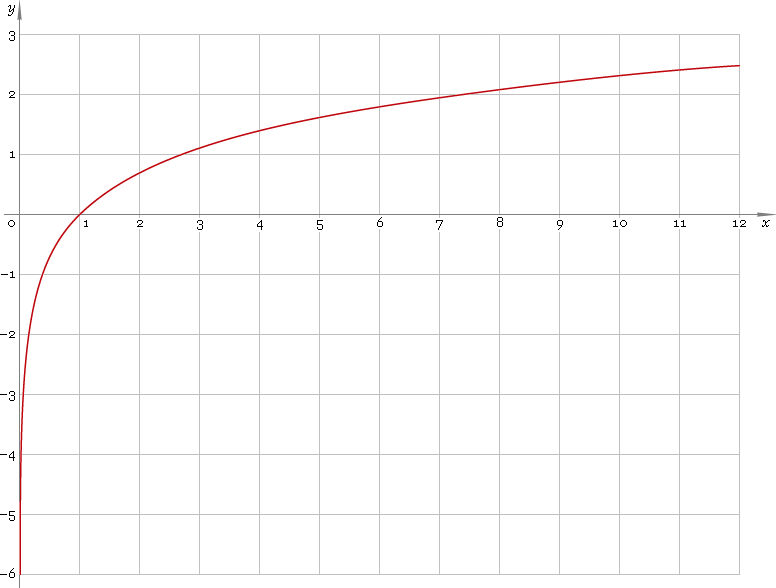The Art of Interface

# ln — natural logarithmic function

Category. Mathematics.

Abstract. Natural logarithm: definition, plot, properties and identities.

## 1. Definition

Natural logarithmic function is the inverse of the exponential function.

## 2. Plot

Natural logarithmic function is defined on positive part of the real axis — so, its domain is (0, +∞). 0 is a singular point. Function plot is depicted below — fig. 1.Fig. 1. Plot of the natural logarithmic function y = lnx.

Function codomain is entire real axis.

## 3. Identities

By definition:

ln exx

Reciprocal argument:

ln(1/x) = −lnx

Product and ratio of arguments:

ln(xy) = lnx + lny
ln(x /y) = lnx − lny

Power of argument:

lnxa = a lnx

Base change:

logax = lnx /lna
logax = logbx /logba

## 4. Support

Natural logarithmic function ln of the real argument is supported by free version of the Librow calculator.

Natural logarithmic function ln of the complex argument is supported by professional version of the Librow calculator.

## 5. How to use

To calculate natural logarithm of the number:

``ln(2);``

To calculate natural logarithm of the current result:

``ln(rslt);``

To calculate natural logarithm of the number x in memory:

``ln(mem[x]);``